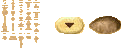computability logic (2003)

Rod Girle's Modal Logics and Philosophy (2000)

Kleine Einführung in die Aussagenlogik (1992) von Marion Soreth

linear logic (1987)

dynamic logic (1970s)

Evert Willem Beth (1970) Formal Methods: An introduction to

symbolic logic and to the study of effective operations in arithmetic and logic

Relational algebra (1970)

fuzzy logic (1965)

abstract algebraic logic (1950s)

Frederic Fitch's Symbolic Logic (1952)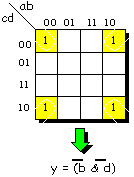Karnaugh maps (1952)Logistics (1950s)

the Nash equilibrium (1950)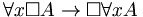the Barcan Formula (1946)

higher-order logic (1940)

topological logic (1937)

Turing machines (1936)

metalogic (1930s)

Lambda calculus (1930s)

model theory (1930s)

infinitary logic (1931)

Heyting (1930) Die formalen Regelen der Intuitionistischen Logik

relevance logic (1928)

Mereology (1927)

deontic logic (1926)

intuitionistic logic (1925)

proof theory (1922)

combinatory logic (1920)

ternary logic (1920)

multi-valued logic (1920)

Polish notation (1920)

p -⊰ q

the strict conditional (1918)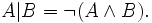the Sheffer stroke (1913)

intuitionistic logic (1912)

modal logic (1910)

Louis Couturat (1904) proposed the name "La logistique" for modern logic

type theory (1903)

Nikola Tesla (1899) invented the electronic AND logic gate circuit

Ernst Schröder's Vorlesungen über die Algebra der Logik (1890-1905) prepared the way for the

emergence of mathematical logic as a separate discipline in the twentieth century by systematizing the

various systems of formal logic of the day

Peano axioms (1889)

mathematical logic (1887)Friedrich Harms' Logik (1886)

Second-order logic (1885)

Peirce's law (1885)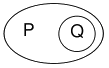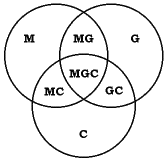Venn diagrams (1881)

the amphecks (1880) were discovered by Charles S. Peirce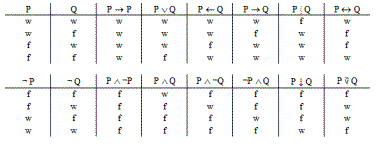truth tables (1880)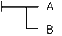Frege's Begriffsschrift (1879)

first-order logic (1879)

Hugh MacColl (1879) published a four-part article setting out the first known variant of the

propositional calculus, calling it the "calculus of equivalent statements", anteceding Frege's Begriffschrifft

set theory (1874)Jevons' Logic Piano (1869)

boolean algebra (1860s)

algebraic logic (1850s)

boolean logic (1854)

which are founded the Mathematical Theories of Logic and Probabilities

Henry Longueville Mansel (1851) Prolegomena logica: an

Inquiry into the Psychological Character of Logical Processes

symbolic logic (1847)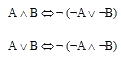De Morgan's laws (1847)

game theory (1838)

digital logic (1837)

logic gates (1837) were first used by Charles Babbage in constructing his Analytical Engine

William Whewell's (1837) History of the Inductive Sciences, from the Earliest to the Present Time

Hegelian dialectic (19th century)

Hegel's Science of Logic (1811-1816)

Gottfried Ploucquet (1782) developed a symbolic calculusEuler diagrams (1768)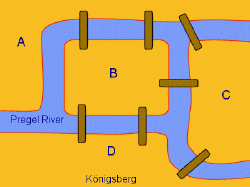the Port-Royal Logic (1662)

Francisco Suarez' Metaphysical Disputations (early seventeenth century)

Cajetan distinguished between the extension and intension of terms (1530)

Rodolphus Agricola (1479) De inventione dialectica

Paul of Venice (15th century) Logica parva et logica magna

Occam's razor (14th-century)

Albert of Saxony (14th century) Perutilis logica, Quaestiones logicales, Quaestiones on the Ars Vetus

Jean Buridan (14th century) Compendium Logicae, Summa de Dialecticâ

William of Ockham (14th century) Summa logicae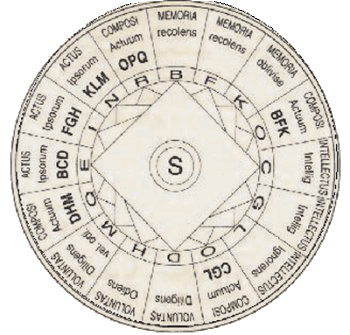Ramon Lull's Ars Magna (1275) contained one of the earliest attempts to construct a universal language

Roger Bacon's Opus majus (1267)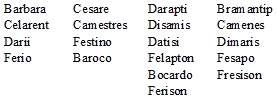Tractatus, Summule logicales magistri Petri Hispani (13th century)

Adam Parvipontanus (12th century) Ars disserendi

John of Salisbury (12th century) Metalogicon

Gilbert de la Porrée (12th century) De sex principiis

Abu Hamid al Ghazali (12th century) composed three works on Aristotelian logic,

Mi'yar al-'ilm (The Standard Measure of Knowledge), Mihakk al-nazar f'l-mantiq

(The Touchstone of Proof in Logic) and al-Qistas al-mustaqim (The Just Balance)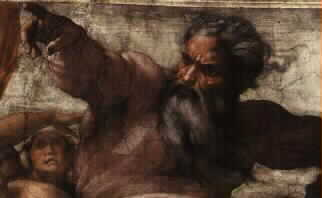Averroës (12th century) was one of the first philosophers to propose the omnipotence paradox

Scholasticism (1100-1500)

the fourth syllogistic figure (Middle Ages) was derived from

the indirect modi of the first figure by Islamic philosophers

Dharmakirti (circa 7th century) Seven Treatises on Valid Cognition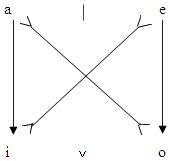Dignaga (5th century) Treatise on the Correct Principles of Logic

Sextus Empiricus' Adversus Mathematicos (3rd century)

Porphyry's Introduction to Categories (late 3rd century)

Galen's Institutio logica (2nd century)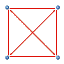the square of opposition was introduced by Apuleius (2nd century)

to illustrate the relations between Aristotle's four basic propositions

the surviving logical works of Aristotle were arranged by Andronicus of Rhodos (40 bc)

into six books, this body was later on called Organon (instrument) by the Byzantine logicians

the five undemonstrated modes of Chrysippus (mid 3rd century bc)

the law of double negation

propositional calculus was developed by the Stoics,

ordinal numerals were used as variables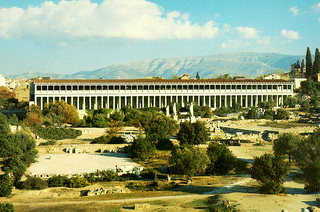Stoic logic (early 3rd century bc)

Zeno of Citium introduced the term "logic" to replace Aristotle`s term "analytic"

Philo of Megara defined material implication with a truth table (late 4th century)

Diodorus' master argument (late 4th century)

Diodorus Chronos invented propositional calculus (late 4th century)

Aristotelian logic (330 bc)

Eubulides of Miletus (4th century bc) is credited with the invention of several logical paradoxes

Plato's Euthydemus (380 bc)

the Megarian school of philosophy (400 bc) was founded by Euclid of Megara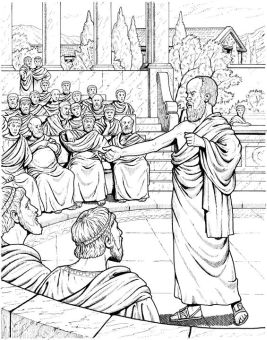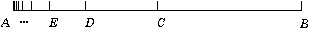Sophistry (fifth century bc)

chinese logic (fifth century bc)

indian logic (sixth century bc)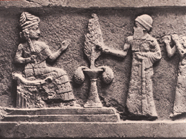legal logic (21st century bc)